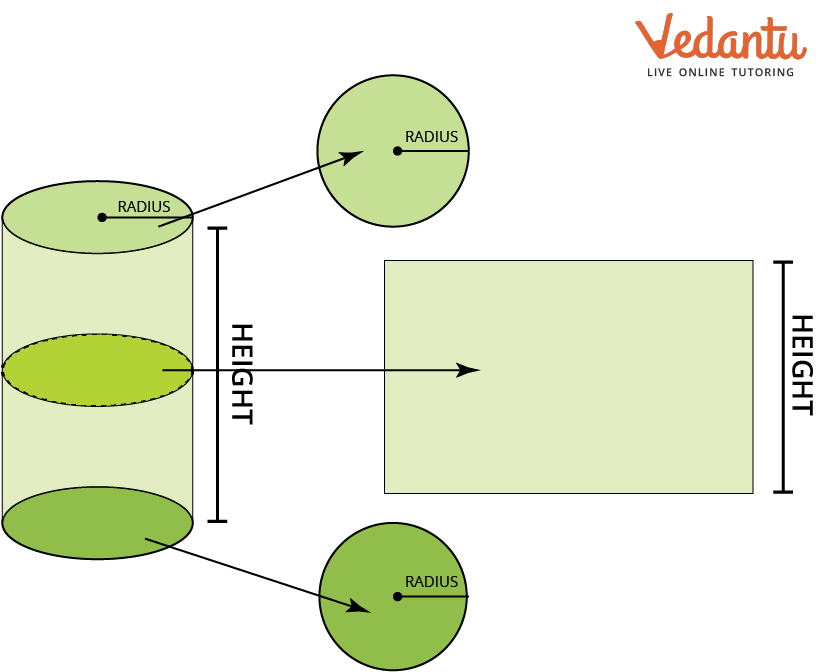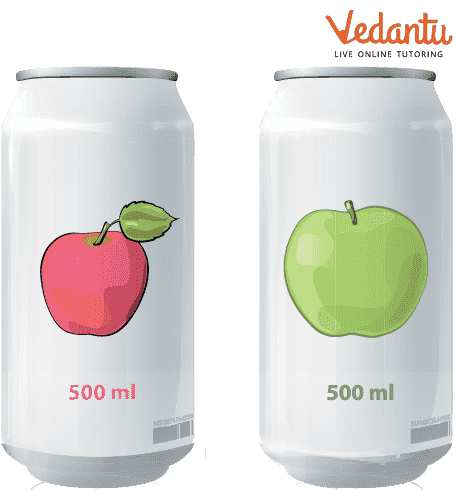Courses
Courses for Kids
Free study material
Free LIVE classes
More

# Problems on Right Circular CylinderLIVE
Join Vedantu’s FREE Mastercalss

## Introduction

Right circular cylinder is a three-dimensional solid figure. On both ends of the right circular cylinder, which are parallel to one another, there is a closed circular surface. The right cylinder is another name for a right circular cylinder. All of the points on the closed surface are equally spaced from the axis of the right circular cylinder. A cylinder has two curved edges, one curved surface and two flat circular faces. In everyday life, a right circular cylinder is the most typical 3D figure.

## What is a Right Circular Cylinder?

Right circular cylinders are three-dimensional solid shapes made up of two parallel bases connected by a closed circular surface, with each base resembling a disk-shaped circle. The axis of the right cylinder is the line that connects the centres of two circular bases or runs through their middles.

Height, or "h," stands for the perpendicular distance, which is the distance between the cylinder's two bases. The radius of the right circular cylinder, denoted by "r," is the measurement from the centre to the outside border for either of the two bases. As a result, two circles and a rectangle combine to create a right circular cylinder.Right Circular Cylinder

## Properties of Right Circular Cylinder

Like any two-dimensional shape, the right circular cylinder possesses the following characteristics:

• It has one curved surface and two flat surfaces.

• Cylindrical bases are always parallel to one another.

• The cylinder's area and volume are determined by its height as well as the radius of its base.

• The bases are perfectly above one another, and the axis makes a right angle with them.

• It lacks a vertex or a corner.

• The cylinder's top and bottom are always exact replicas of one another.

• The axis is the line that connects the circle's centres.

• A right cylinder is created when a rectangle is rotated around one side as the axis of rotation.

• The rectangle is the portion that results from cutting a right circular cylinder with a plane that has two components and runs parallel to the cylinder's axis.

• A circle is formed when a plane cuts the right cylinder horizontally and parallel to the bases.

## Surface Area of Right Circular Cylinder

The area that the right circular cylinder's surface covers is referred to as the surface area of the right circular cylinder. A right circular cylinder's surface regions may be divided into two types:

### 1. Curved Surface Area of Right Circular Cylinder

It is also known as the right circular cylinder's lateral surface area. It is the space between the parallel circular bases of the right circular cylinder, which is covered by the curved surface of the cylinder.

The formula for curved surface area is

Curved Surface Area (CSA) = $2\pi rh$ square units.

Where “r” is the radius and “h” is the height of the cylinder.

### 2. Total Surface Area of Right Circular Cylinder

It is described as the region that the complete cylinder fills. There are two circles and a curved surface in this region of the cylinder. So, in order to calculate the right circular cylinder's total surface area, we sum the areas of the two circles and the curved surface at the end.

The total surface area is given as:

Total Surface Area (TSA) = $2\pi r h + 2\pi {r^2} = 2\pi r(h + r)$ square units.

Where “r” is the radius and “h” is the height of the cylinder.

## Volume of Right Circular Cylinder

The quantity of space occupied by a right circular cylinder is referred to as its volume. In terms of cubic units, the volume of the right circular cylinder is expressed as (m3, cm3, in3, or ft3).

The formula for volume of right circular cylinder is:

Volume of cylinder = Area of Circle x Height

Volume = $\pi {r^2} h$ cubic units

Where “r” is the radius and “h” is the height of the cylinder.

## Cylinder Examples in Real Life

From soda cans to toilet paper to water pipelines, cylinders are everywhere in our life. Some examples of cylinders are:

• Water tanks

• Candles

• Battery

• Test tube

• BeakersJuice Cans

## Problems on Right Circular Cylinders

Let us look at some question on cylinder which will also include volume of a cylinder problems and cylinder word problem:

Example 1: The volume of a right circular cylinder is 7040 in3. If its radius is 4 in, find its height. (use $\pi = \dfrac{{22}}{7}{\rm{ ~or~ }}3.14$)

Solution: Volume of cylinder = $440~i{n^3}$

To find the height, the formula to be used is

$\begin{array}{l}V = \pi {r^2} \times h\\7040 = \dfrac{{22}}{7} \times {4^2} \times h\\h = \dfrac{{7040 \times 7}}{{22 \times 16}}\\h = 140~in\end{array}$

Hence, the height of the cylinder is 140 in.

Example 2: Find the volume of a right cylinder, if the radius and height of the cylinder are 20 cm and 30 cm respectively. (use $\pi = \dfrac{{22}}{7}{\rm{ ~or~ }}3.14$)

Solution:

Given: r = 20 cm, h = 30 cm

Volume of the cylinder is given as:

$\begin{array}{l}V = \pi {r^2}h\\V = 3.14 \times {20^2} \times 30\\V = 37680~c{m^3}\end{array}$

Hence, the volume of the cylinder is 37680 cm3.

## Conclusion

We now have a basic understanding of the right circular cylinder and its key components due to this article. This article covered topics like surface area and volume of the right circular cylinder. Properties of the right circular cylinder is also discussed and now we have a good knowledge about the circular cylinder. The problems related to cylinders can be solved using the formulas discussed in this article. Some real life examples of circular cylinders are also mentioned.

Last updated date: 26th Sep 2023
Total views: 84.3k
Views today: 1.84k

## FAQs on Problems on Right Circular Cylinder

1. What is the difference between cylinder and right circular cylinder?

Right circular cylinder definition states that- A cylinder whose base is a circle is called a circular cylinder. If the axis of the cylinder is perpendicular to its base then the cylinder is called a right circular cylinder.

2. How is the right circular cylinder formed?

Rolling the rectangle on one of its sides and using that side as the axis of the cylinder creates an object known as a right circular cylinder. If the axis (one of the rectangle's sides) is perpendicular to the radius, the cylinder created in this fashion is referred to as a right circular cylinder (r).

3. What is the axis of a cylinder?

This cylinder's axis runs through the centre of the circle and is perpendicular to the circle's plane. The axis's angle with the circle in an oblique circular cylinder is different from 90°.